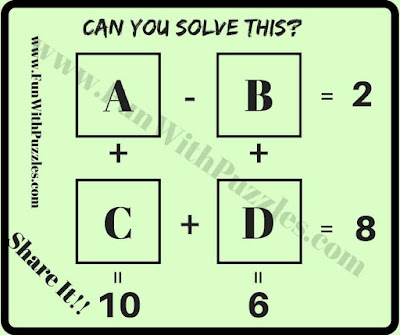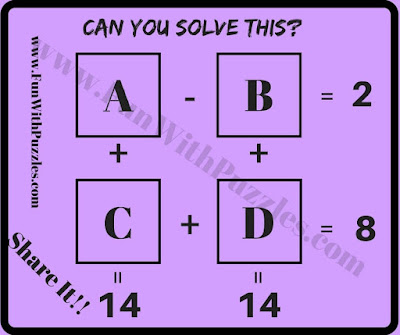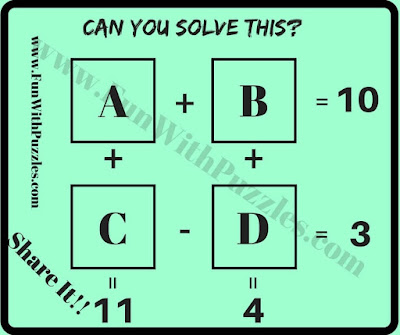Mathematical is a very interesting subject. Students will love Maths if this subject is thought in an interesting way. Here we are presenting few Mathematical equation puzzles which are presented in the form of picture puzzles. These are easy Mathematical Questions for students. Let us see how many of these equations you can solve it quickly?
How to Solve Mathematical Puzzle Questions
In each of these Easy Mathematical Puzzle Questions, four Mathematical equations are given as Picture Puzzle. There are four variables in these equations marked as A, B, C and D. Your challenge is to solve these equations and get the values of these variables as quickly as possible.
The answer to these Mathematical Puzzles Questions is given at the end.1. Can you solve these Mathematical Equations?2. Can you find values of A, B, C and D in these equations?3. What are the values of A, B, C and D in these equations?4. Solve these Mathematical Equations5. Will you solve these Mathematical Equations?

Do check out below mentioned Maths Brain Teasers and Puzzles to challenge your brain
List of Mathematical Brain Teasers and Puzzles

1. Math Brain Teasers With Answers: It contains many easy Maths Brain Teasers for Kids and Teens to twist your mind.

2. Math Picture Brain Teasers with Answers and Explanation: It contains different types of Mathematical Brain Teasers presented in the form of Picture Puzzles Images.

3. Middle School Math Brain Teasers: It contains Fun Maths Brain Teasers for Students to challenge your brain.

4. Number Brain Teasers: It contains Mathematical Puzzles in which number equations contains the logical patterns. Your challenge is to find these logical patterns and then find the value of the number which will replace the question mark.

Unknown said...

puzzle 4 answer is supposed to be: 5 4
3 2

Fun With Puzzles said...

Unknown said...

Fist Puzzle answer is A=5 B=3 C=5 and D=3

Rajesh Kumar said...

Unknown said...

your answer is right but this answer is also right if a= 6, b=3, c=2 and d=3

Unknown said...

2ND EQUATION A=9 B=2 C=6 D=5 1st EQUATION A=11 B=9 C=3 D=5

Unknown said...

Puzzel 4 ans
4+5=9
4+1=5
8=6

Unknown said...

2nd Equation A=11 B=9 C=3 D=5 this is the proper ans

Unknown said...

A=5,B=3,C=5,D=3

Bhoumya Ranjan Mantry said...

Answer of 5 is A = 7,B = 3, C=4, D=1

Sari said...

A=9,B=2, C=6, D=5

Unknown said...

Plzz give me solution of 1st puzzle
A=5 B=3 C=5 D=3

Unknown said...

Bro plzz give me.solution of 1st puzzle plzzz

Rajesh Kumar said...

You can check out the answers of all these maths puzzles by clicking on the answer link in this post.

Unknown said...

Answer for the 3rd puzzle is=
A=6
B=5
C=9
D=2